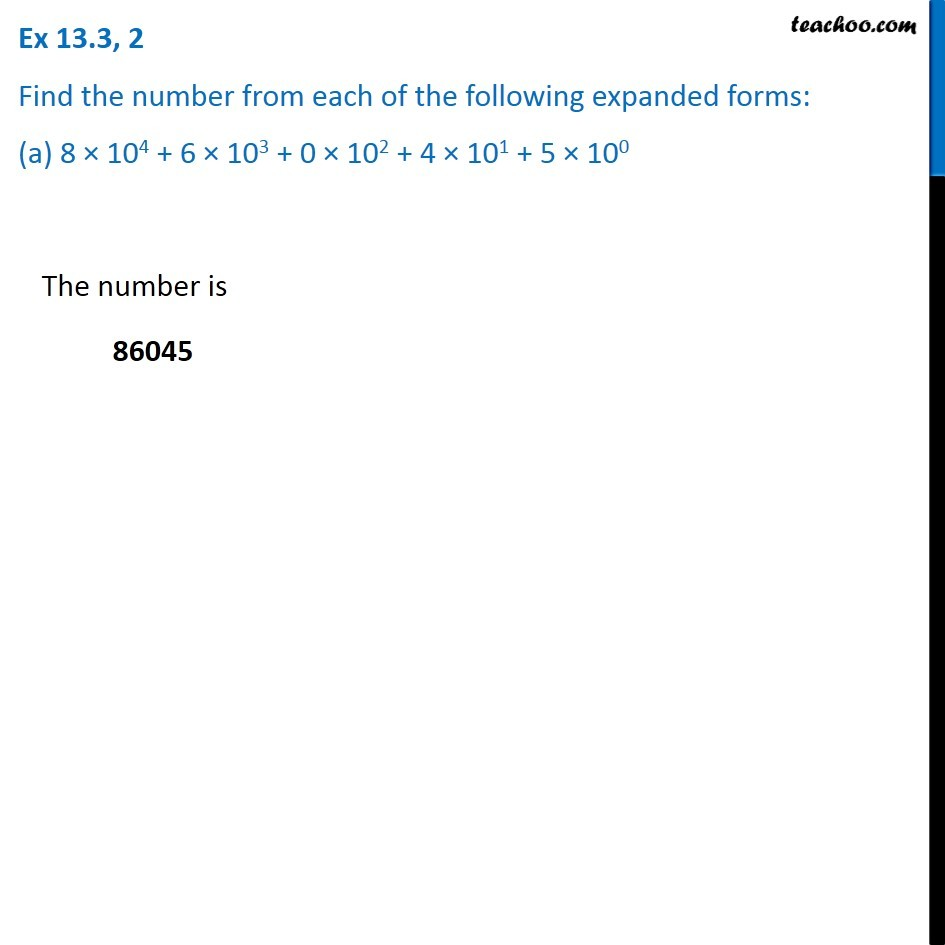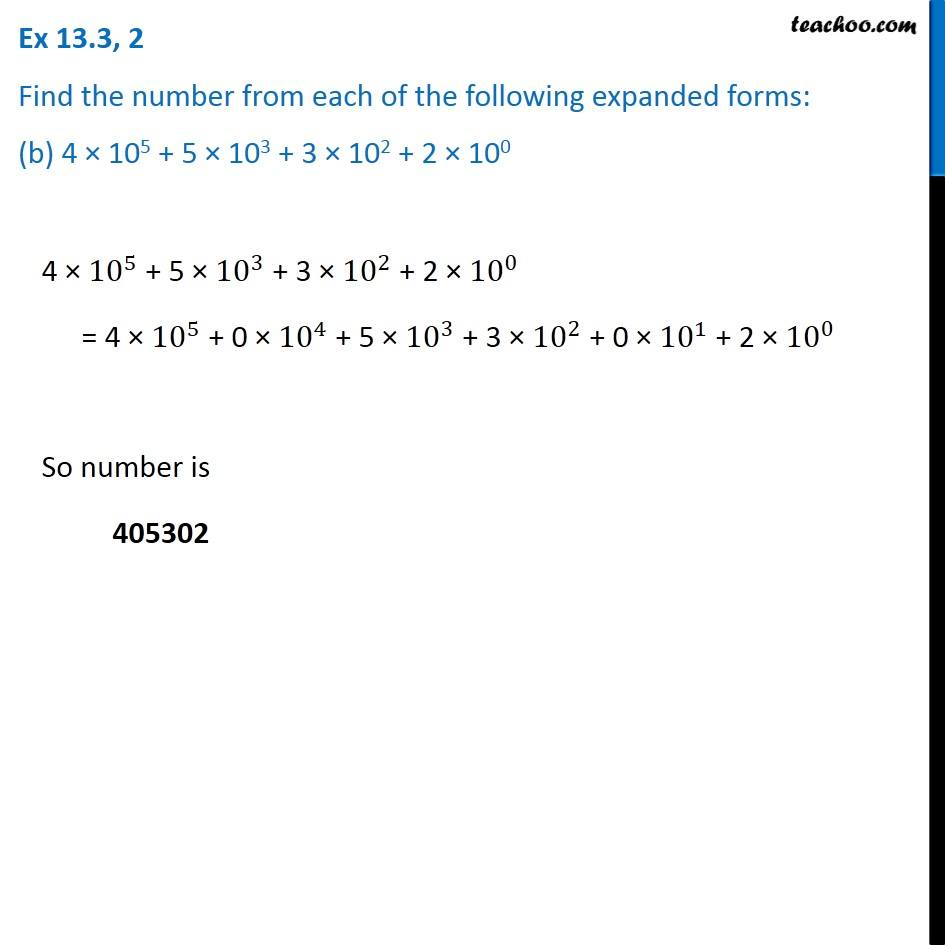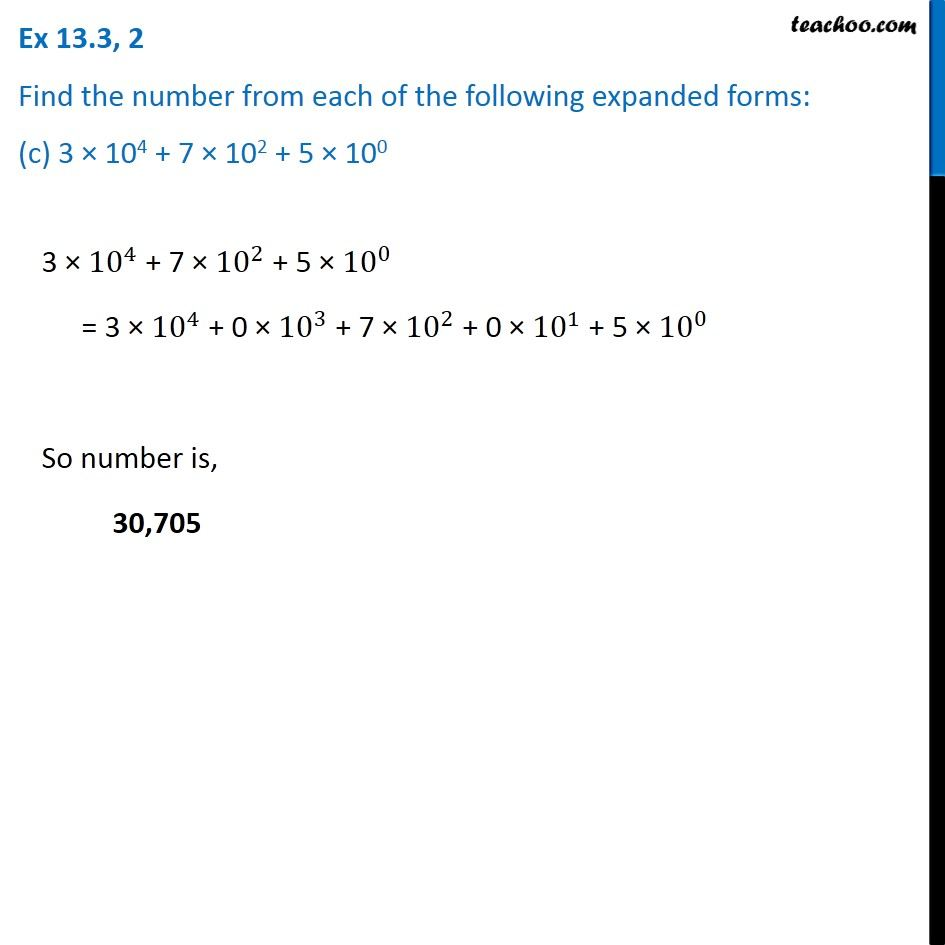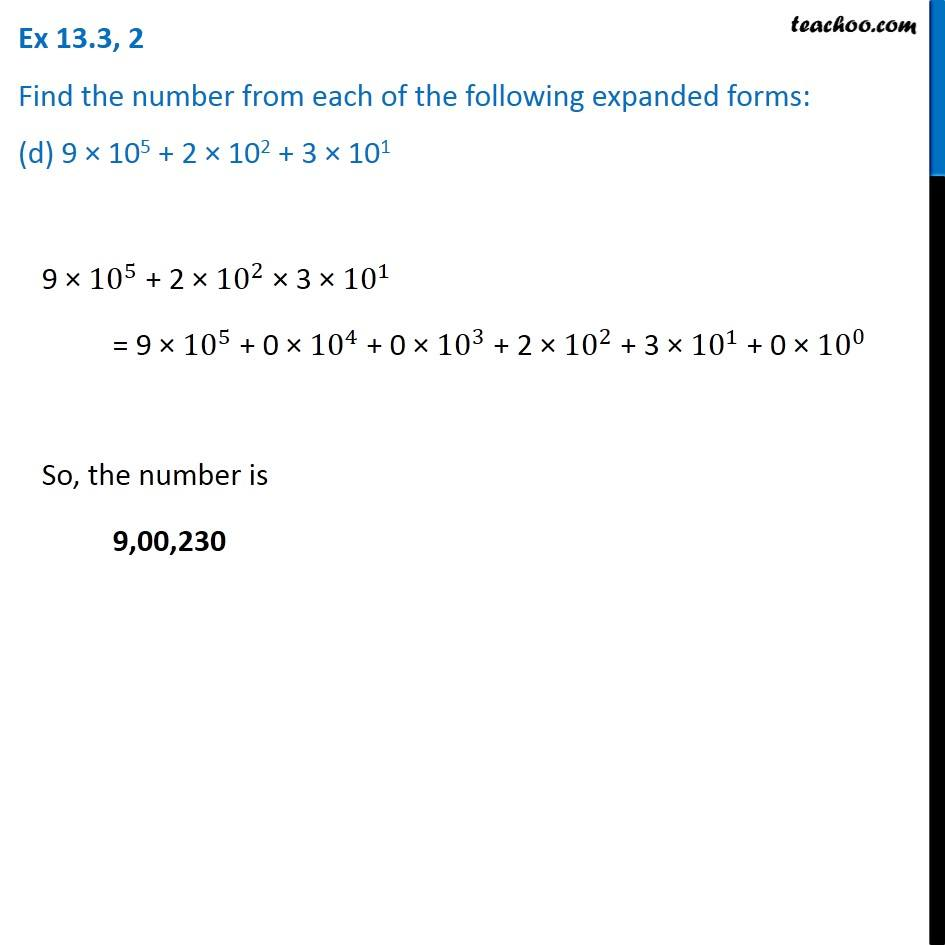Subscribe to our Youtube Channel - https://you.tube/teachoo

1. Chapter 13 Class 7 Exponents and Powers
2. Serial order wise
3. Ex 13.3

Transcript

Ex 13.3, 2 Find the number from each of the following expanded forms: (a) 8 × 104 + 6 × 103 + 0 × 102 + 4 × 101 + 5 × 100 The number is 86045 Ex 13.3, 2 Find the number from each of the following expanded forms: (b) 4 × 105 + 5 × 103 + 3 × 102 + 2 × 100 4 × 〖10〗^5 + 5 × 〖10〗^3 + 3 × 〖10〗^2 + 2 × 〖10〗^0 = 4 × 〖10〗^5 + 0 × 〖10〗^4 + 5 × 〖10〗^3 + 3 × 〖10〗^2 + 0 × 〖10〗^1 + 2 × 〖10〗^0 So number is 405302 Ex 13.3, 2 Find the number from each of the following expanded forms: (c) 3 × 104 + 7 × 102 + 5 × 100 3 × 〖10〗^4 + 7 × 〖10〗^2 + 5 × 〖10〗^0 = 3 × 〖10〗^4 + 0 × 〖10〗^3 + 7 × 〖10〗^2 + 0 × 〖10〗^1 + 5 × 〖10〗^0 So number is, 30,705 Ex 13.3, 2 Find the number from each of the following expanded forms: (d) 9 × 105 + 2 × 102 + 3 × 101 9 × 〖10〗^5 + 2 × 〖10〗^2 × 3 × 〖10〗^1 = 9 × 〖10〗^5 + 0 × 〖10〗^4 + 0 × 〖10〗^3 + 2 × 〖10〗^2 + 3 × 〖10〗^1 + 0 × 〖10〗^0 So, the number is 9,00,230

Ex 13.3